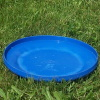#### You may also like### Master Minding

Your partner chooses two beads and places them side by side behind a screen. What is the minimum number of guesses you would need to be sure of guessing the two beads and their positions?### Flippin' Discs

Discs are flipped in the air. You win if all the faces show the same colour. What is the probability of winning?### Odds and Evens

Are these games fair? How can you tell?

# At Least One...

##### Age 11 to 14Challenge Level

We received a large number of responses of excellent quality.

Ben from St Peter's followed the tree diagram and calculated out the answer:

If you flip a coin three times the chance of getting at least one head is 87.5%. To get this outcome I used the provided tree diagram to establish how many outcomes used one head.

Llewellyn from St Peter's and Diamor from Willington County Grammar School both observed an interesting pattern and expanded the answer to flipping ten coins:

If you flip a coin 3 times the probability of getting at least one heads is 7 in 8 by reading the table. This table also works the opposite way, the chances of Charlie getting no heads is 1 in 8 because out of all the outcomes only one of them has only tails. I notice that if you add these probabilities together you get the total amount of outcomes (7+1=8). If you flip a coin 4 times the probability of you getting at least one heads is 15 in 16 because you times the amount of outcomes you can get by flipping 3 coins by 2, it results in 16 and then you minus 1 from it. With 5 coins to flip you just times 16 by 2 and then minus 1, so it would result with a 31 in 32 chance of getting at least one heads. With 6 coins you times by 2 and minus by 1 again resulting in a 63 in 64 chance. To find the chance of getting at least one heads if you flip ten coins you times 64 by 2 four times or by 16 once and then minus 1, this results in a 1063 in 1064 chance of getting at least one heads.

Neeraj from Wilson School developed a generalization for different numbers of possible outcomes:

I noticed that when you add the probabilities together they make a whole. A quick way of figuring out how many times you get at least one head is, that it is always the no. Of possible outcomes minus one over the no. of possible outcomes So: if No of possible outcomes = n the equation would be: P= (n- 1)/n

One student suggested how to calculate the number of desired outcomes:

If the number of times flipped =p Then the number of outcomes that contain a head is$2^p-1$
So for flipping a coin $10$ times, the number of outcomes with at least one head is $2^{10}-1 = 1024 - 1 = 1023$

Luke from Maidstone Grammar School went further to investigate the next part of the question:

When there are 4 green balls in the bag and there are 6 red balls the probability of randomly selecting a green ball is 0.4 ($\frac{2}{5}$) and the probability selecting a red ball is 0.6 ($\frac{3}{5}$).

If a ball is selected and then replaced the probability of picking a red ball or a green ball is the same every time. When 3 balls are picked with replacement the probability of getting at least one green is
1-(the probability of getting 3 reds)
Because the probability is the same every time the chance of getting 3 reds is $0.6^3=0.216$ (or in fractions $(\frac{3}{5})^3 = \frac{27}{125}$). So the probability of getting at least one green is $1-0.216=0.784$ (or in fractions $1 - \frac{27}{125} = \frac{98}{125}$).

When the balls are not replaced the probability of getting at least one green is still 1-(the probability of getting 3 reds). In each draw the probability of drawing a red ball is $\frac{\text{the number of red balls}}{\text{the total number of balls}}$
On the first draw there are 6 red balls out of 10 so the probability of picking a red is $\frac{6}{10}$.
On the second draw there are 5 red balls out of 9 so the probability of picking a red is $\frac{5}{9}$.
On the final draw there are 4 red balls out of 8 so the probability of picking a red is $\frac{4}{8}$.
The probability of this sequence of draws happening is the probability of each draw multiplied together. i.e.: $\frac{6}{10}\times\frac{5}{9}\times\frac{4}{8}=\frac{1}{6}$
The probability of drawing all reds is $\frac{1}{6}$ and so the probability of drawing at least one green is $\frac{5}{6}$.

Helen from Stroud finished up the problem:

When children are selected for the school council they are not replaced. The children are selected one after another and each time the probability of a boy being selected is
P(boy selected first) = $\frac{\text{the number of boys in the class}}{\text{the total number of children in the class}}$
Note: the class refers to students who have not already been made part of the council.
To find the probability that there will be at least one boy, find the probability that all three are girls, and then
P(at least one boy selected) = 1-P(all girls selected)
P(girl selected first) = $\frac{\text{number of girls in class}}{\text{total number in class}}= \frac{15}{28}$
Then P(second selected also a girl) = $\frac{14}{27}$
And P(third selected also a girl) = $\frac{13}{26}$
So P(all girls selected) = $\frac{15}{28}\times\frac{14}{27}\times\frac{13}{26} = \frac{5}{36}$
P(at least one boy selected) = 1 - P(all girls selected) = 1 - $\frac{5}{36}$ = $\frac{31}{36}$